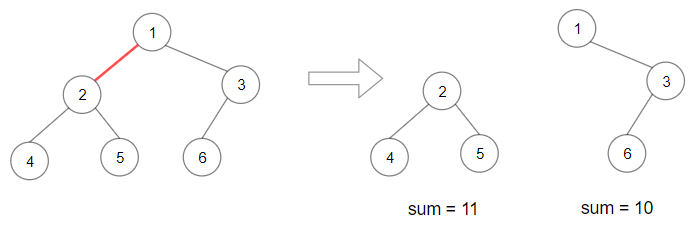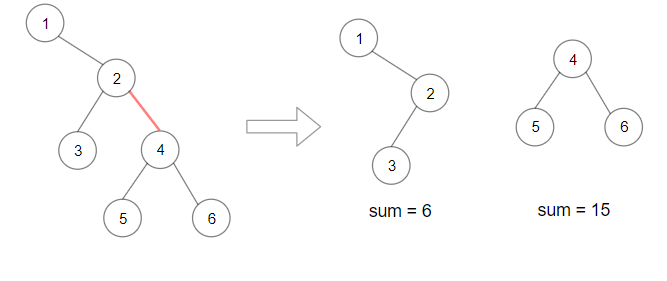# 1339. Maximum Product of Splitted Binary Tree ⭐

Medium
Given the `root` of a binary tree, split the binary tree into two subtrees by removing one edge such that the product of the sums of the subtrees is maximized.
Return the maximum product of the sums of the two subtrees. Since the answer may be too large, return it modulo `109 + 7`.
Note that you need to maximize the answer before taking the mod and not after taking it.
Example 1:Input: root = [1,2,3,4,5,6]
Output: 110
Explanation: Remove the red edge and get 2 binary trees with sum 11 and 10. Their product is 110 (11*10)
Example 2:Input: root = [1,null,2,3,4,null,null,5,6]
Output: 90
Explanation: Remove the red edge and get 2 binary trees with sum 15 and 6.Their product is 90 (15*6)
Constraints:
• The number of nodes in the tree is in the range `[2, 5 * 10^4]`.
• `1 <= Node.val <= 10^4`

### 解題

/**
* Definition for a binary tree node.
* type TreeNode struct {
* Val int
* Left *TreeNode
* Right *TreeNode
* }
*/
func maxProduct(root *TreeNode) int {
// 先求 sum
sum := sum(root)
ans := 0
var helper func(*TreeNode) int
helper = func(root *TreeNode) int {
if root == nil { return 0 }
sumLeft := helper(root.Left)
sumRight := helper(root.Right)
ans = max(ans, max(sumLeft * (sum - sumLeft), sumRight * (sum - sumRight)))
return sumLeft + sumRight + root.Val
}
helper(root)
return ans % 1000000007
}
func sum(root *TreeNode) int {
if root == nil {
return 0
}
return root.Val + sum(root.Left) + sum(root.Right)
}
func max(a, b int) int {
if a > b { return a }
return b
}